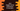# What is Jagged Arrays in Java : explanation with examplesJagged arrays are two-dimensional arrays. You can think like each element of these arrays have elements of type Array, i.e. it holds different arrays. The size of these array-elements is different. It is not required that all elements should have the same sized array.

Jagged arrays are also known as Ragged arrays in Java. In this tutorial, we will learn different ways to create a Jagged array in Java and different examples to understand it better.

### Creating a Jagged Array :

So, the Jagged Array is nothing but an array of arrays. So, we can create it like other arrays. If the elements of the array are known to us, we can declare and initialize the array in one step like below :

``int[][] myArray = {{1,2,3},{4,5},{6}};``

Here, we have created one Jagged array of three elements. Each element is an array of integers. First element is {1,2,3}, second element is {4,5},and the third element is {6}.In this case, we know the elements of the array. So, it becomes easier for us to create the array. If we don’t know the elements, then we need to declare it first and initialize it later. Suppose, we know that the array will hold 3 elements , but don’t know what are they. Then, we will first declare it like below :

``int[][] myArray = new int[];``

After the declaration, we can initialize its first, second and third elements as below :

``````myArray = new int[]{1,2,3,4};
myArray = new int[]{5,6};
myArray = new int[]{7};``````

### Read and store elements in a dynamic sized Jagged array :

We can create different sized or dynamic sized Jagged array. Each element of the array can be dynamic i.e. the size will be different for each array element. Below program will show you how to create one dynamic sized array by taking the size of the array from the user :

``````import java.util.Scanner;

class Main {
public static void main(String[] args) {
int sizeOfArray; //1
Scanner scanner;

scanner = new Scanner(System.in); //2
System.out.println("How many elements your array will hold : ");
sizeOfArray = scanner.nextInt(); //3

int[][] myArray = new int[sizeOfArray][]; //4

for (int i = 0; i < sizeOfArray; i++) { //5
System.out.println("Enter element count for column " + (i + 1));
int count = scanner.nextInt();
myArray[i] = new int[count];    //6

for (int j = 0; j < count; j++) {
System.out.println("Enter element " + (j + 1)); //7
myArray[i][j] = scanner.nextInt();
}
}
}
}``````

### Explanation :

The commented numbers in the above program denote the step number below :

1. We are not declaring the size of the array. sizeOfArray variable is initialized to store the size.
2. Create one Scanner object to read user inputs.
3. Ask the user to enter the size of the array. Read the number and store it in sizeOfArray.
4. Now, create the Jagged array same as user input size.
5. Run one for loop. This loop will run for the size of the array times. Each time ask the user how many elements are there for that specific row.
6. Read the element count and store it in count variable. Create one integer array of size count for that particular row.
7. Similarly, run one for loop, ask the user to enter an element for that row and read all the array elements for all the rows.

This program will read all elements for a Jagged array and store it in an array object. The output of the program will look like as below :

``````How many elements your array will hold :
3
Enter element count for column 1
2
Enter element 1
1
Enter element 2
2
Enter element count for column 2
3
Enter element 1
1
Enter element 2
2
Enter element 3
3
Enter element count for column 3
1
Enter element 1
56``````

The above example will create the below array :

``{{1,2},{1,2,3},{56}}``

### How to print the elements of a Jagged array :

Since the Jagged array is actually a 2D array, we can use two for loops to print all the contents of the array. Below example will show you how to print the elements of a Jagged array using two nested for loops :

``````class Main {
public static void main(String[] args) {
int jaggedArray[][] = {{1, 2, 3}, {4, 5}, {6, 8, 9}};

for (int i = 0; i < jaggedArray.length; i++) {
System.out.println("Elements stored in position " + i);
for (int j = 0; j < jaggedArray[i].length; j++) {
System.out.print(" " + jaggedArray[i][j]);
}
System.out.println("\n");
}
}
}``````

It will print the below output :

``````Elements stored in position 0
1 2 3

Elements stored in position 1
4 5

Elements stored in position 2
6 8 9``````

### Conclusion :

In the example above, we have learnt what is a Jagged array and how to read and print out the contents of a Jagged array. The jagged array is useful for organizing similar data in an application. You can try the above example problem and if you have any question, please drop one comment below.